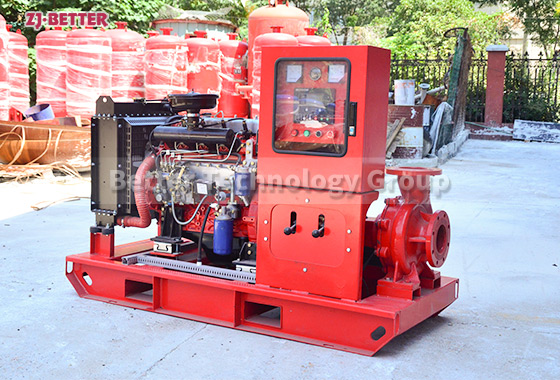What is the meaning of "flow" in fire pump?

2023-06-30

The meaning of pump flow:
The flow rate of the pump is the amount of water delivered by the pump per unit time. The flow rate on the pump nameplate is the design flow rate, also known as the rated flow rate. The water pump has high efficiency when it operates at rated flow and rated head. When using a water pump, the water output of the water pump should be consistent with or close to the rated flow.
Units of pump flow:
There are generally three time units: seconds, minutes, and hours. In my country, flow units adopt international metric units, such as liters, milliliters, cubic meters, thousand cubic meters, etc., and different units are used according to different usage environments.
Expressed by Q, in terms of l/s, m3/s, m3/h. ...
Unit m3/h: It is the number of cubic meters of internal flow per hour.
T is ton, 1T=1m3, so 1T/H=1m3/h is a flow unit (cubic meter or ton per hour), and L/S is also a flow unit, that is (liter/second=L/S). 1m3/h=1000L/3600S=1L/3.6S=0.28L/SThere are many factors affecting the pump flow rate, which can be divided into two categories: objective factors and subjective factors.
The objective factor is the rated flow rate selected by the user. The larger the rated flow rate is selected, the larger the actual working flow rate will be. The factory flow rate of the water pump is consistent with the performance parameters of the water pump.
many subjective factors:
①For example, the model of submersible sewage pump selected by the customer is: WQ65-25-30-7.5. The user's water distribution pipe is DN40mm. The flow rate of the pump becomes smaller when the pump is working. The flow will decrease accordingly. Assuming that the actual head used is 35 meters, the flow rate will also decrease during work.
②When the user is installing, there are many bends formed by the pipeline, which will also reduce the flow rate of the pump.
③Insufficient water intake of the pump is also a factor affecting the flow rate.
④ If there are branch pipes on the main pipe, the flow rate will also become smaller.
⑤ There is debris blocking the inlet and outlet pipes.
⑥ voltage instability or voltage phase loss.
⑦ impeller wear and so on.WhatsApp me